# WDM System Performance Issues

The most important issue in the design of WDM lightwave systems is the interchannel crosstalk. The system performance degrades whenever crosstalk leads to transfer of power from one channel to another. Such a transfer can occur because of the nonlinear effects in optical fibers, a phenomenon referred to as nonlinear crosstalk as it depends on the nonlinear nature of the communication channel. However, some crosstalk occurs even in a perfectly linear channel because of the imperfect nature of various WDM components such as optical filters, demultiplexers, and switches. In this section we discuss both the linear and nonlinear crosstalk mechanisms and also consider other performance issues relevant for WDM systems.

#### 1. Heterowavelength Linear Crosstalk

Linear crosstalk can be classified into two categories depending on its origin. Optical filters and demultiplexers often let leak a fraction of the signal power from neighboring channels that interferes with the detection process. Such crosstalk is called heterowavelength or out-of-band crosstalk and is less of a problem because of its incoherent nature than the homowavelength or in-band crosstalk that occurs during routing of the WDM signal from multiple ports. This subsection focuses on the heterowavelength crosstalk.

Consider the case in which a tunable optical filter is used to select a single channel among the N channels incident on it.  If the optical filter is set to pass the mth channel, the optical power reaching the photodetector can be written as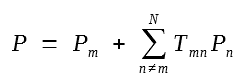where Pm is the power in the mth channel and Tmn is the filter transmittivity for channel n when channel m is selected. Crosstalk occurs if Tmn ≠ 0 for n ≠ m. It is called out-of-bank crosstalk because it belongs to the channels lying outside the spectral band occupied by the channel detected. Its incoherent nature is also apparent from the fact that it depends only on the power of the neighboring channels.

To evaluate the impact of such crosstalk on system performance, one should consider the power penalty, defined as the additional power required at the receiver to counteract the effect of crosstalk. The photocurrent generated in response to the incident optical power is given by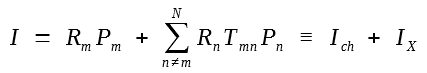where Rm = ηmq/hνm is the photodetector responsivity for channel m at the optical frequency νm and ηm is the quantum efficiency. The second term IX  denotes the crosstalk contribution to the receiver current I. Its value depends on the bit pattern and becomes maximum when all interfering channels carry 1 bits simultaneously (the worst case).

A simple approach to calculating the crosstalk power penalty is based on the eye closure occurring as a result of the crosstalk. The eye closes most in the worst case for which IX is maximum. In practice, Ich is increased to maintain the system performance. If Ich needs to be increased by a factor δX, the peak current corresponding to the top of the eye is I1 = δXIch + IX. The decision threshold is set at ID = I1/2. The eye opening from ID to the top level would be maintained at its original value Ich/2 ifor when δX = 1 + IX/Ich. The quantity δX is just the power penalty for the mth channel. By using IX and Ich from the previous equation, δX can be written (in dB) aswhere the powers correspond to their on-state values. If the peak power is assumed to be the same for all channels, the crosstalk penalty becomes power independent. Further, if the photodetector responsivity is nearly the same for all channels (Rm ≈ Rn), δX is well approximated by

δX = 10log10(1 + X)

whereis a measure of the out-of-band crosstalk; it represents the fraction of total power leaked into a specific channel from all other channels. The numerical value of X depends on the transmission characteristics of the specific optical filter. For a FP filter, X can be obtained in a closed form.

The preceding analysis of crosstalk penalty is based on the eye closure rather than the bit-error rate (BER). One can obtain an expression for the BER if IX is treated as a random variable. For a given value of IX, the BER is obtained by using the analysis of the previous tutorial. In particular, the BER is given with the on- and off-state currents given by I1 = Ich + IX and  I0 = IX if we assume that Ich = 0 in the off-state. The decision threshold is set at ID = Ich(1 + X)/2, which corresponds to the worst-case situation in which all neighboring channels are in the on state. The final BER is obtained by averaging over the distribution of the random variable IX. The distribution of IX has been calculated for a FP filter and is generally far from being Gaussian. The crosstalk power penalty δX can be calculated by finding the increase in Ich needed to maintain a certain value of BER. The following figure shows the calculated penalty for several values of BER plotted as a function of N/F with the choice F = 100. The solid curve corresponds to the error-free case (BER = 0). The power penalty can be kept below 0.2 dB to maintain a BER of 10-9 for values of N/F as large as 0.33. The channel spacing can be as little as three times the bit rate for such FP filters.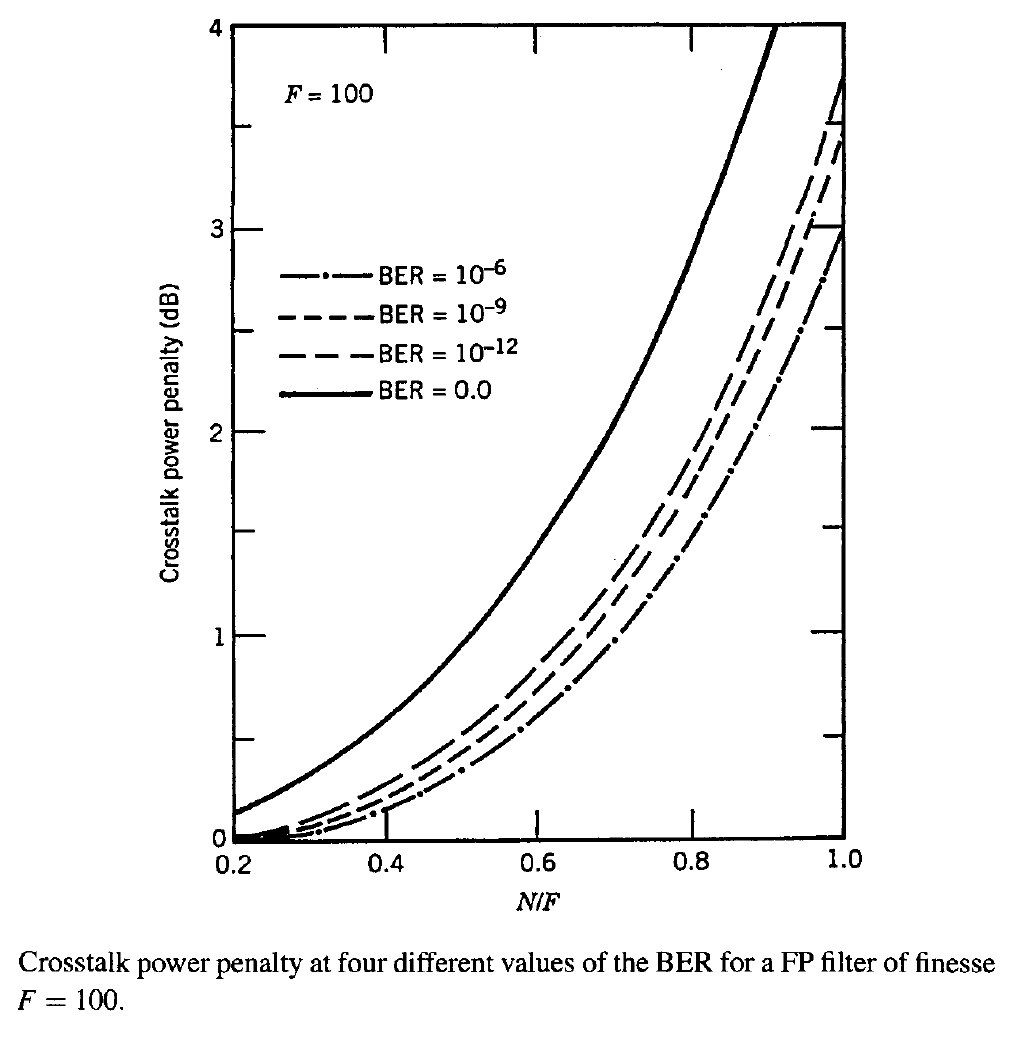#### 2. Homowavelength Linear Crosstalk

Homowavelength or in-band crosstalk results from WDM components used for routing and switching along an optical network and has been of concern since the advent of WDM systems. Its origin can be understood by considering a static wavelength router such as a WGR shown below.For an N x N router, there exists N2 combinations  through which N-wavelength WDM signals can be split. Consider the output at one wavelength, say λm. Among the N2 -1 interfering signals that can accompany the desired signal, N - 1 signals have the same carrier wavelength λm, while the remaining N(N - 1) belong to different carrier wavelengths and are likely to be eliminated as they pass through other WDM components. The N - 1 crosstalk signals at the same wavelength (in-band crosstalk) originate from incomplete filtering through a WGR because of its partially overlapping transmission peaks. The total optical field, including only the in-band crosstalk, can be written aswhere Em is the desired signal and ωm = 2πc/λm. The coherent nature of the in-band crosstalk is obvious from this equation.

To see the impact of in-band crosstalk on system performance, we should again consider the power penalty. The receiver current I = R|Em(t)|2 in this case contains interference or beat terms similar to the case of optical amplifiers.  One can identify two types of beat terms; signal-crosstalk beating with terms like EmEn and crosstalk-crosstalk beating with terms like EkEn, where k ≠ m and n ≠ m. The latter terms are negligible in practice and can be ignored. The receiver current is then given approximately as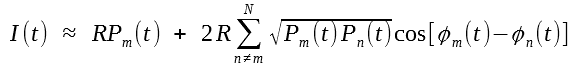where Pn = |En|2 is the power and φn(t) is the phase. In practice, Pn << Pm for n ≠ m because a WGR is built to reduce the crosstalk. Since phases are likely to fluctuate randomly, we can write the above equation as I(t) = R(Pm + ΔP), treat the crosstalk as intensity noise. We then obtain the power penalty as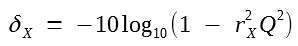whereand X = Pn/Pm is the crosstalk level defined as the fraction of power leaking through the WGR and is taken to be the same for all N - 1 sources of coherent in-band crosstalk by assuming equal powers. An average over the phases was performed by replacing cos2θ = 1/2. In addition, rX2 was multiplied by another factor of 1/2 to account for the fact that Pn is zero on average half of the times (during 0 bits). Experimental measurements of power penalty for a WGR agree with this simple model.

To keep the power penalty below 2 dB, rX < 0.07 is required, a condition that limits X(N - 1) to below -23 dB. Thus, the crosstalk level X must be below -38 dB for N = 16 and below -43 dB for N = 100, rather stringent requirements.

The calculation of crosstalk penalty in the case of dynamic wvelength routing through optical cross-connects becomes quite complicated because of a large number of crosstalk elements that a signal can pass through in such WDM networks. The worst-case analysis predicts a large power penalty (> 3 dB) when the number of crosstalk elements becomes more than 25 even if the crosstalk level of each component is only -40 dB. Clearly, the linear crosstalk is of primary concern in the design of WDM networks and should controlled. Several techniques have been proposed for this purpose.

#### 3. Nonlinear Raman Crosstalk

Several nonlinear effects in optical fibers can lead to interchannel and intrachannel crosstalk that affects the system performance considerably. We discussed such nonlinear effects and their origin from a physical point of view. This subsection focuses on the Raman crosstalk.

As discussed before, stimulated Raman scattering (SRS) is generally not of concern for single-channel systems because of its relatively high threshold (about 500 mW near 1.55 μm). The situation is quite different for WDM systems in which the fiber acts as a Raman amplifier such that the long-wavelength channels are amplified by the short-wavelength channels as long as the wavelength difference is within the bandwidth of the Raman gain. The Raman gain spectrum of silica fibers is so broad that amplification can occur for channels spaced as far apart as 100 nm. The shortest-wavelength channel is most depleted as it can pump many channels simultaneously. Such an energy transfer among channels can be detrimental for system performance as it depends on the bit pattern - amplification occurs only when 1 bits are present in both channels simultaneously. The Raman-induced crosstalk degrades the system performance and is of considerable concern for WDM systems.

Raman crosstalk can be avoided if channel powers are made so small that SRS-induced amplification is negligible over the entire fiber length. It is thus important to estimate the limiting value of the channel power. A simple model considers the depletion of the highest-frequency channel in the worst case in which 1 bits of all channels overlap completely simultaneously. The amplification factor for each channel is Gm = exp(gmLeff), where Leff is the effective interaction length and gm = gRm)Pch/Aeff is the Raman gain at Ωm = ω1 - ωm. For gmLeff << 1, the shortest-wavelength channel at ω1 is depleted by a fraction gmLeff due to Raman amplification of the mth channel. The total depletion for a M-channel WDM system is given byThe summation in this equation can be carried out analytically if the Raman gain spectrum is approximated by a triangular profile such that gR increases linearly for frequencies up to 15 THz with a slope SR = dgR/dν and then drops to zero. Using gRm) = mSRΔνch, the fractional power loss for the shortest-wavelength channel becomes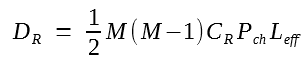where CR = SRΔνch/(2Aeff). In deriving this equation, channels were assumed to have a constant spacing Δνch and the Raman gain for each channel was reduced by a factor of 2 to account for random polarization states of different channels.

A more accurate analysis should consider not only depletion of each channel because of power transfer to longer-wavelength channels but also its own amplification by shorter-wavelength channels. If all other nonlinear effects are neglected along with GVD, evolution of the power Pn associated with the nth channel is governed by the following equation: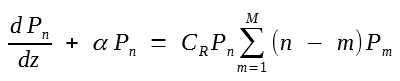where α is assumed to be the same for all channels. This set of M coupled nonlinear equations can be solved analytically. For a fiber of length L, the result is given bywhereis the total input power in all channels. This equation shows that channel powers follow an exponential distribution because of Raman-induced coupling among all channels.

The depletion factor DR for the shorter-wavelength channel (n = 1) is obtained using DR = (P10 - P1)/P10, where P10 = P1(0)exp(-αL) is the channel power expected in the absence of SRS. In the case of equal input powers in all channels, Pt = MPch and DR is given by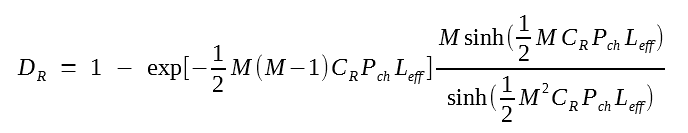In the limit M2CRPchLeff << 1, this complicated expression reduces to the simple result:In general, this simple equation overestimates the Raman crosstalk.

The Raman-induced power penalty is obtained using δR = -10log(1 - DR) because the input channel power must be increased by a factor of (1 - DR)-1 to maintain the same system performance. The figure below shows how the power penalty increases with an increase in the channel power and the number of channels.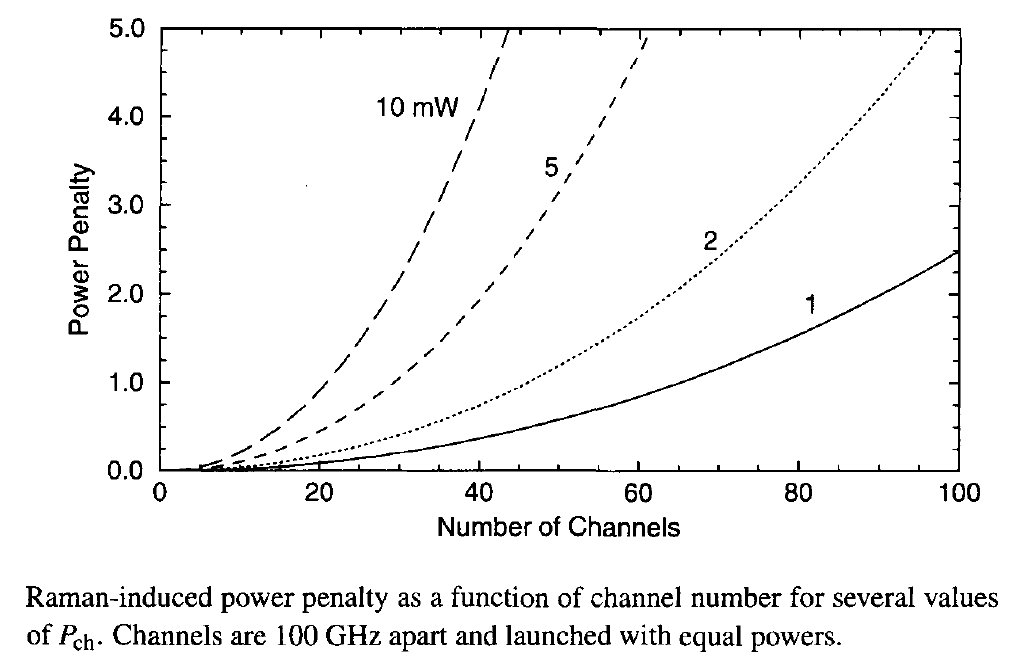The channel spacing is assumed to be 100 GHz. The slope of the Raman gain is estimated from the gain spectrum to be SR = 4.9 x 10-18 m/(W-GHz) while Aeff = 50 μm2 and Leff ≈ 1/α = 21.74 km. As seen from the figure, the power penalty becomes quite large for WDM systems with a large number of channels. If a value of at most 1 dB is considered acceptable, the limiting channel power Pch exceeds 10 mW for 20 channels, but its value is reduced to below 1 mW when the number of WDM channels is larger than 70.

The foregoing analysis provides only a rough estimate of the Raman crosstalk as it neglects the fact that signals in each channel consist of a random sequence of 0 and 1 bits. A statistical analysis shows that the Raman crosstalk is lower by about a factor of 2 when signal modulation is taken into account. The GVD effects that were neglected in the above analysis also reduce the Raman crosstalk since pulses in different channels travel at different speeds because of the group-velocity mismatch. On the other hand, periodic amplification of the WDM signal can magnify the impact of SRS-induced degradation. The reason is that in-line amplifiers add noise which experiences less Raman loss than the signal itself, resulting in degradation of the SNR. The Raman crosstalk under realistic operating conditions was calculated in a 2003 study. Numerical simulations showed that it can be reduced by inserting optical filters along the fiber link that block the low-frequency noise below the longest-wavelength channel. Raman crosstalk can also be reduced using the technique of midway spectral inversion.

#### 4. Stimulated Brillouin Scattering

Stimulated Brillouin scattering (SBS) can also transfer energy from a high-frequency channel to a low-frequency one when the channel spacing equals the Brillouin shift. However, in contrast with the case of SRS, such an energy transfer is easily avoided with the proper design of multichannel communication systems. The reason is that the Brillouin-gain bandwidth is extremely narrow (~ 20 MHz) compared with the Raman-gain bandwidth (~ 5 THz). Thus, the channel spacing must match almost exactly the Brillouin shift (about 10 GHz in the 1.55-μm region) for SBS to occur; such an exact match is easily avoided. Furthermore, the two channels must be counterpropagating for Brillouin amplification to occur.

Although SBS does not induce interchannel crosstalk when all channels propagate in the forward direction, it nonetheless limits the channel powers. The reason is that a part of the channel power can be transferred to a backward-propagating Stokes wave generated from noise when the threshold condition gBPthLeff/Aeff ≈ 21 is satisfied. This condition is independent of the number and the presence of other channels. However, the threshold for each channel can be reached at low power levels. The figure below shows how the output power and power reflected backward through SBS vary in a 13-km-long dispersion-shifted fiber as the injected CW power is increased from 0.5 to 50 mW. No more than 3 mW could be transmitted through the fiber in this experiment after the Brillouin threshold. For a fiber with Aeff = 50 μm2 and α = 0.2 dB/km, the threshold power is below 2 mW when the fiber length is long enough (> 20 km) that Leff can be replaced by 1/α.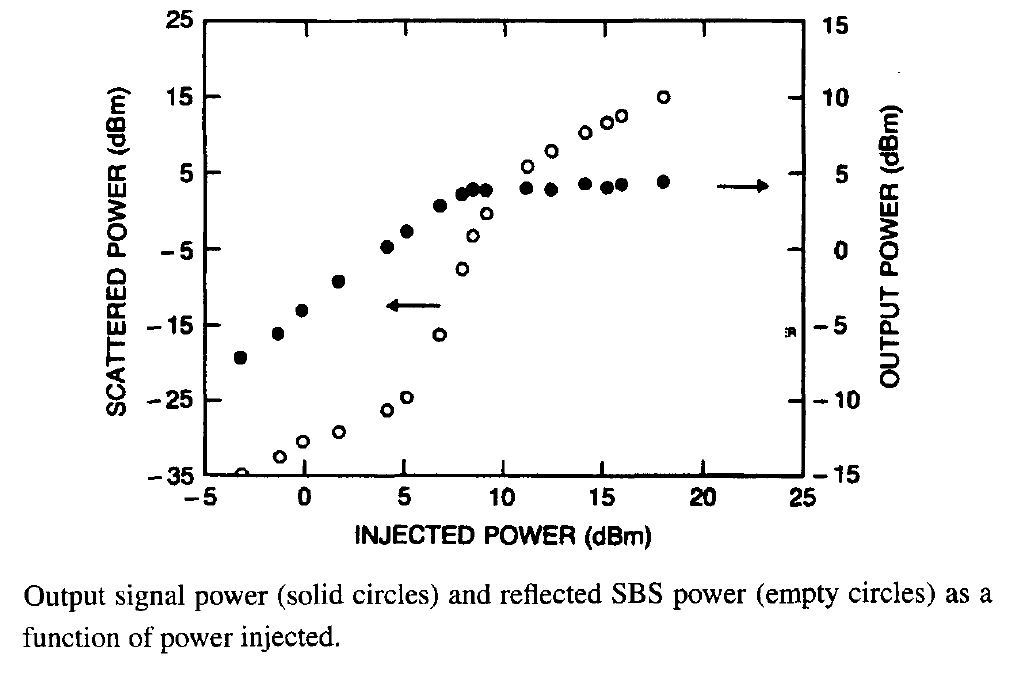The preceding estimate applies to CW signals as it neglects the effects of signal modulation resulting in a random sequence of 0 and 1 bits. In general, the Brillouin threshold depends on the modulation format as well as on the ratio of the bit rate to the Brillouin-gain bandwidth. It increases to above 5 mW  for lightwave systems operating near 10 Gb/s. Some applications require launch powers in excess of 10 mW. Several schemes have been proposed for raising the Brillouin threshold. They rely on increasing either the Brillouin-gain bandwidth ΔνB or the spectral width of optical carrier. The former has a value of about 20 MHz for silica fibers, while the latter is typically < 10 MHz for DFB lasers used in WDM systems. The bandwidth of an optical carrier can be increased without affecting the system performance by modulating its phase at a frequency much lower than the bit rate. Typically, the modulation frequency Δνm is in the range of 200-400 MHz. As the effective Brillouin gain is reduced by a factor (1 + Δνm/ΔνB), the SBS threshold increases by the same factor. Since typically ΔνB ~ 20 MHz, the launched power can be increased by more than a factor of 10 by this technique.

If the Brillouin-gain bandwidth ΔνB of the fiber itself can be increased from its nominal value of 20 MHz to more than 200 MHz, the SBS threshold can be increased without requiring a phase. One technique sues sinusoidal strain along the fiber length for this purpose. The applied strain changes the Brillouin shift νB by a few percent in a periodic manner. The resulting Brillouin-gain spectrum is much broader than that occurring for a fixed value of νB. The strain can be applied during cabling of the fiber. In one fiber cable, ΔνB  was found to increase from 50 to 400 MHz. The Brillouin shift νB can also be changed by making the core radius nonuniform along the fiber length since the longitudinal acoustic frequency depends on the core radius. The same effect can be realized by changing the dopant concentration along the fiber length. This technique increased the SBS threshold of one fiber by 7 dB. A side effect of varying the core radius or the dopant concentration is that the GVD parameter β2 also changes along the fiber length. It is possible to vary both of them simultaneously in such a way that β2 remains relatively uniform. Phase modulation induced by a supervisory channel through the nonlinear phenomenon of cross-phase modulation (XPM) can also be used to suppress SBS. XPM induced by neighboring channels can also help, but it is hard to control and is itself a source of crosstalk. In practice, a frequency modulator integrated within the transmitter provides the best method for suppressing SBS. Threshold levels > 200 mW have been realized with this technique.

#### 5. Cross-Phase Modulation

Teh SPM and XPM both affect the performance of WDM systems. The effects of SPM has been discussed in previous tutorials in the context of single-channel system; they also apply to individual channels of a WDM system. The phenomenon of XPM is an important mechanism of nonlinear crosstalk in WDM lightwave systems and has been studied extensively in this context.

XPM originates from the intensity dependence of the refractive index, which produces an intensity-dependent phase shift as the signal propagates through the optical fiber. The phase shift for a specific channel depends not only on the power of that channel but also on the power of other channels. The total phase shift for the jth channel is given by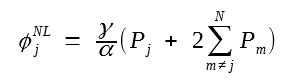where the first term is due to SPM and Leff has been replaced with 1/α assuming αL >> 1. The parameter γ is in the range 1-10 W-1km-1 depending on the type of fiber used, larger values occurring for dispersion-compensating fibers. The nonlinear phase shift depends on the bit pattern of various channels and can vary from zero to its maximum value φmax = (γ/α)(2N - 1)Pj for N channels, if we assume equal channel powers.

Strictly speaking, the XPM_induced phase shift should not affect system performance if the GVD effects were negligible. However, any dispersion in fiber converts pattern-dependent phase shifts to power fluctuations, reducing the SNR at the receiver. This conversion can be understood by noting that time-dependent phase changes lead to frequency chirping that affects dispersion-induced broadening of the signal. The figure below shows XPM-induced fluctuations for a CW probe launched with a 10-Gb/s pump channel modulated using the NRZ format. The probe power fluctuates by as much as 6% after 320 km of dispersive fiber. The root-mean-square (RMS) value of fluctuations depends on the channel power and can be reduced by lowering it. As a rough estimate, it we use the condition φmax < 1, the channel power is restricted to

Pch < α/[γ(2N - 1)]

For typical values of α and γ, Pch should be below 10 mW even for five channels and reduces to below 1 mW for more than 50 channels.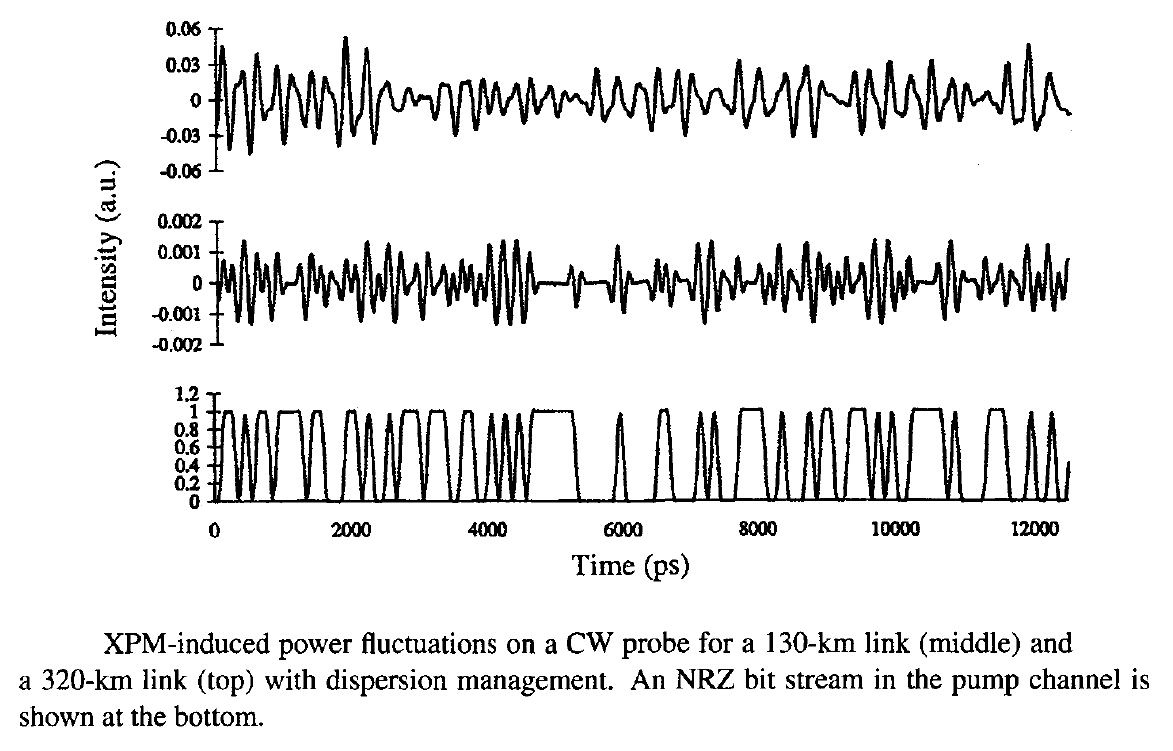The preceding analysis provides only a rough estimate as it ignores the fact that pulses belonging to different channels travel at different speeds and walk through each other at a rate that depends on their wavelength difference. Since XPM can occur only when pulses overlap in the time domain, its impact is reduced considerably by the wall-off effects. As a faster-moving pulse belonging to one channel collides with and passes through a specific pulse in another channel, the XPM-induced chirp shifts the pulse spectrum first toward red and then toward blue. In a lossless fiber, collisions of two pulses are perfectly symmetric, resulting in no net spectral shift at the end of the collision. In a loss-managed system with optical amplifiers placed periodically along the link, power variations make collisions between pulses of different channels asymmetric, resulting in a net frequency shift that depends on the channel spacing. Such frequency shifts lead to timing jitter (the speed of a channel depends on its frequency because of GVD) since their magnitude depends on the bit pattern as well as on the channel wavelengths. The combination of XPM-induced amplitude and timing jitter degrades the SNR at the receiver, especially for closely spaced channels, and leads to XPM-induced power penalty that depends on channel spacing and the type of fibers used for the WDM link. The power penalty increases for fibers with large GVD and for WDM systems designed with a small channel spacing and can exceed 5 dB even for 100-GHz spacing.

How can one control the XPM-induced crosstalk in WDM systems? Clearly, the use of low-GVD fibers will reduce this problem to some extent but is not practical because of the onset of Four-Wave-Mixing (FWM). In practice, dispersion management is employed in virtually all WDM systems such that the local dispersion is relatively large. Careful selection of the dispersion-map parameters may help from the XPM standpoint but may not be optimum from the SPM point of view. A simple approach to XPM suppression consists of introducing relative time delays among the WDM channels after each map period such that the "1" bits in neighboring channels are unlikely to overlap most of the time. The use of RZ format is quite helpful in this context because all 1 bits occupy only a fraction of the bit slot. In a 10-channel WDM experiment, time delays were introduced by using 10 fiber gratings spaced apart by varying distances chosen to enhance XPM suppression. The BER floor observed after 500 km of transmission disappeared after the XPM suppressors (consisting of 10 Bragg gratings) were inserted every 100 km. The residual power penalty at a BER of 10-10 was below 2 dB for all channels.

#### 6. Four-Wave Mixing

The nonlinear phenomenon of FWM requires phase matching. It  becomes a major source of nonlinear crosstalk whenever the channel spacing and fiber dispersion are small enough to satisfy the phase-matching condition approximately. This is the case when a WDM system operates close to the zero-dispersion wavelength of dispersion-shifted fibers. For this reason, several techniques have been developed for reducing the impact of FWM in WDM systems.

The physical origin of FWM-induced crosstalk and the resulting system degradation can be understood by noting that FWM generates a new wave at the frequency ωijk = ωi + ωj - ωk, whenever three waves at frequencies ωi, ωj, and ωk copropagate inside the fiber. For an N-channel system, i, j, and k can vary from 1 to N, resulting in a large combination of new frequencies generated by FWM. In the case of equally spaced channels, the new frequencies coincide with the existing frequencies, leading to coherent in-band crosstalk. When channels are not equally spaced, most FWM components fall in between the channels and lead to incoherent out-of-band crosstalk. In both cases, the system performance is degraded because of a loss in the channel power, but the coherent crosstalk degrades system performance much more severely.

The FWM process in optical fibers is governed by a set of four coupled equations whose general solution requires a numerical approach. If we neglect the phase shifts induced by SPM and XPM, assume that the three channels participating in the FWM process remain nearly undepleted, and include fiber losses, the amplitude AF of the FWM component at the frequency ωF is governed by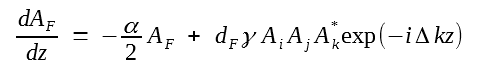where Am(z) = Am(0)exp(- αz/2) for m = i, j, k and dF = 2 - δij is the degeneracy factor defined such that its value is 1 when i = j but doubles when i ≠ j. This equation can be easily integrated to obtain AF(z). The power transferred to the FWM component in a fiber of length L is given bywhere Pm = |Am(0)|2 is the launched power in the mth channel and ηF is a measure of the FWM efficiency defined asThe FWM efficiency ηF depends on the channel spacing through the phase mismatch governed by

Δk = βF + βk - βi - βj ≈ β2i - ωk)(ωj - ωk)

where the propagation constants were expanded in a Taylor series around ωc = (ωi + ωj)/2 and β2 is the GVD parameter at that frequency. If the GVD of the transmission fiber is relatively large, (|β2| > 5 ps2/km),  ηF nearly vanishes for typical channel spacings of 50 GHz or more. In contrast, ηF ≈ 1 close to the zero-dispersion wavelength of the fiber, resulting in considerable power in the FWM component, especially at high channel powers. In the case of equal channel powers, PF increases as Pch3. This cubic dependence of the FWM component limits the channel powers to below 1 mW if FWM is nearly phase matched. Since the number of FWM components for an M-channel WDM system increases as M2(M - 1)/2, the total power in all FWM components can be quite large.

A simple scheme for reducing the FWM-induced degradation consists of designing WDM systems with unequal channel spacings. The main impact of FWM in this case is to reduce the channel power. This power depletion results in a power penalty this is relatively small compared with the case of equal channel spacings. Experimental measurements on WDM systems confirm the advantage of unequal channel spacings. In a 1999 experiment, this technique was used to transmit 22 channels, each operating at 10 Gb/s, over 320 km of dispersion-shifted fiber with 80-km amplifier spacing. Channel spacings ranged from 125 to 275 GHz in the 1532- to 1562-nm wavelength region and were determined using a periodic allocation scheme. The zero-dispersion wavelength of the fiber was close to 1548 nm, resulting in near phase matching of many FWM components. Nonetheless, the system performed quite well with less than 1.5-dB power penalty for all channels.

The use of nonuniform channel spacing is not always practical because many WDM components, such as optical filters and waveguide-grating routers, require equal channel spacings. A practical solution is offered by the periodic dispersion-management technique. In this case, fibers with normal and anomalous GVD are combined to form a dispersion map such that GVD is high locally all along the fiber even though its average value is quite low. As a result, the FWM efficiency ηF is negligible throughout the fiber, resulting in little FWM-induced crosstalk. The use of dispersion management is common for suppressing FWM in WDM systems because of its practical simplicity. In fact, new kinds of fibers, called nonzero-dispersion-shifted fibers (NZDSFs), were designed and marketed after the advent of WDM systems. Typically, GVD is in the range of 4 to 8 ps/(km-nm) in such fibers to ensure that the FWM-induced crosstalk is minimized.

#### 7. Other Design Issues

The design of WDM communication systems requires careful consideration of many transmitter and receiver characteristics. An important issue concerns the stability of the carrier frequency (or wavelength) associated with each channel. The frequency of light emitted from DFB or DBR semiconductor lasers can change considerably because of changes in the operating temperature (~ 10 GHz/°C).  Similar changes can also occur with the aging of lasers. Such frequency changes are generally not of concern for single-channel systems. In the case of WDM lightwave systems it is important that the carrier frequencies of all channels remain stable, at least relatively, so that the channel spacing does not fluctuate with time.

A number of techniques have been used for frequency stabilization. A common technique uses electrical feedback provided by a frequency discriminator using an atomic or molecular resonance to lock the laser frequency to the resonance frequency. For example, one can use ammonia, krypton, or acetylene for semiconductor lasers operating in the 1.55-μm region, as all three have resonances near that wavelength. Frequency stability to within 1 MHz can be achieved by this technique. Another technique makes use of the optogalvanic effect to lock the laser frequency to an atomic or molecular resonance. A phase-locked loop can also be used for frequency stabilization. In another scheme, a Michelson interferometer, calibrated by using a frequency-stabilized master DFB laser, provides a set of equally spaced reference frequencies. A FP filter, an AWG, or any other filter with a comb-like periodic transmission spectrum can also be used for this purpose because it provides a reference set of equally spaced frequencies. A fiber grating is useful for frequency stabilization but a separate grating is needed for each channel as its reflection spectrum is not periodic.

The following figure shows a DFB laser module in which both the optical power and the wavelength of the laser are monitored and maintained at constant values.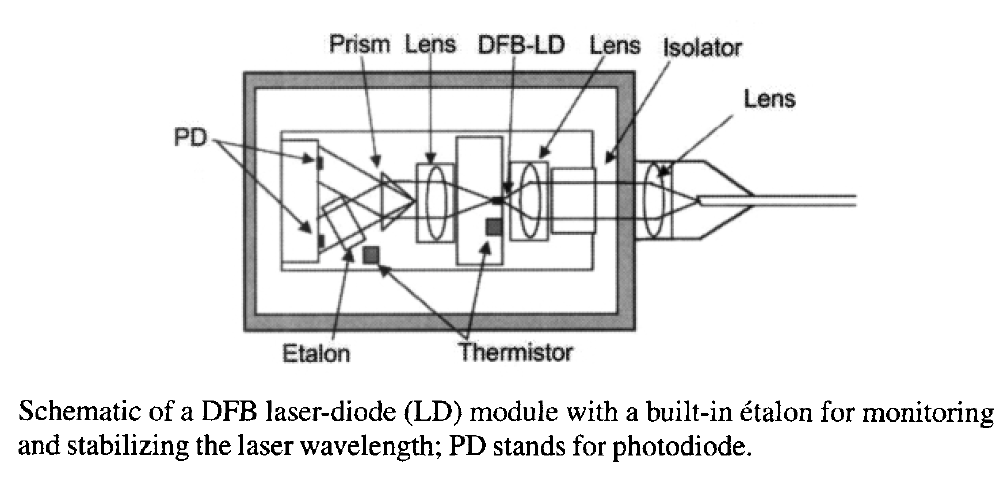Light from the back facet of the DFB laser is split into two branches using a prism. An FP etalon in one branch serves as a wavelength reference; it is designed such that one of its transmission peaks occurs precisely at the wavelength at which the laser is designed to operate. The use of FP etalon as a wavelength reference suffers from one problem. Variations in the etalon temperature can shift its transmission peaks in an uncontrolled manner. This problem is solved by monitoring the temperature of the etalon and adjusting the feedback signal accordingly. With this approach, the wavelength of the laser module drifted by less than 1 pm even when the module temperature varied from 5 to 70°C. Reliability tests indicated that wavelength drift for such lasers should be less than 5 pm during a 25-year operating period.

An important issue in the design of WDM networks is related to the loss of signal power that occurs because of insertion, distribution, and transmission losses. Optical amplifiers are used to compensate for such losses but not all channels are amplified by the same factor unless the gain spectrum is flat over the entire bandwidth of the WDM signal. Although gain-flattening techniques are commonly employed, channel powers can still deviate by 10 dB or more when the WDM signal passes through many optical amplifiers before being detected. It may then become necessary to control the power of individual channels (through selective attenuation) at each node within a WDM network to make the channel powers nearly uniform. The issue of power management in WDM networks is quote complex and requires attention to many details. The buildup of amplifier noise can also become a limiting factor when the WDM signal passes through a large number of amplifiers. In general, management of a WDM network requires attention to many details.